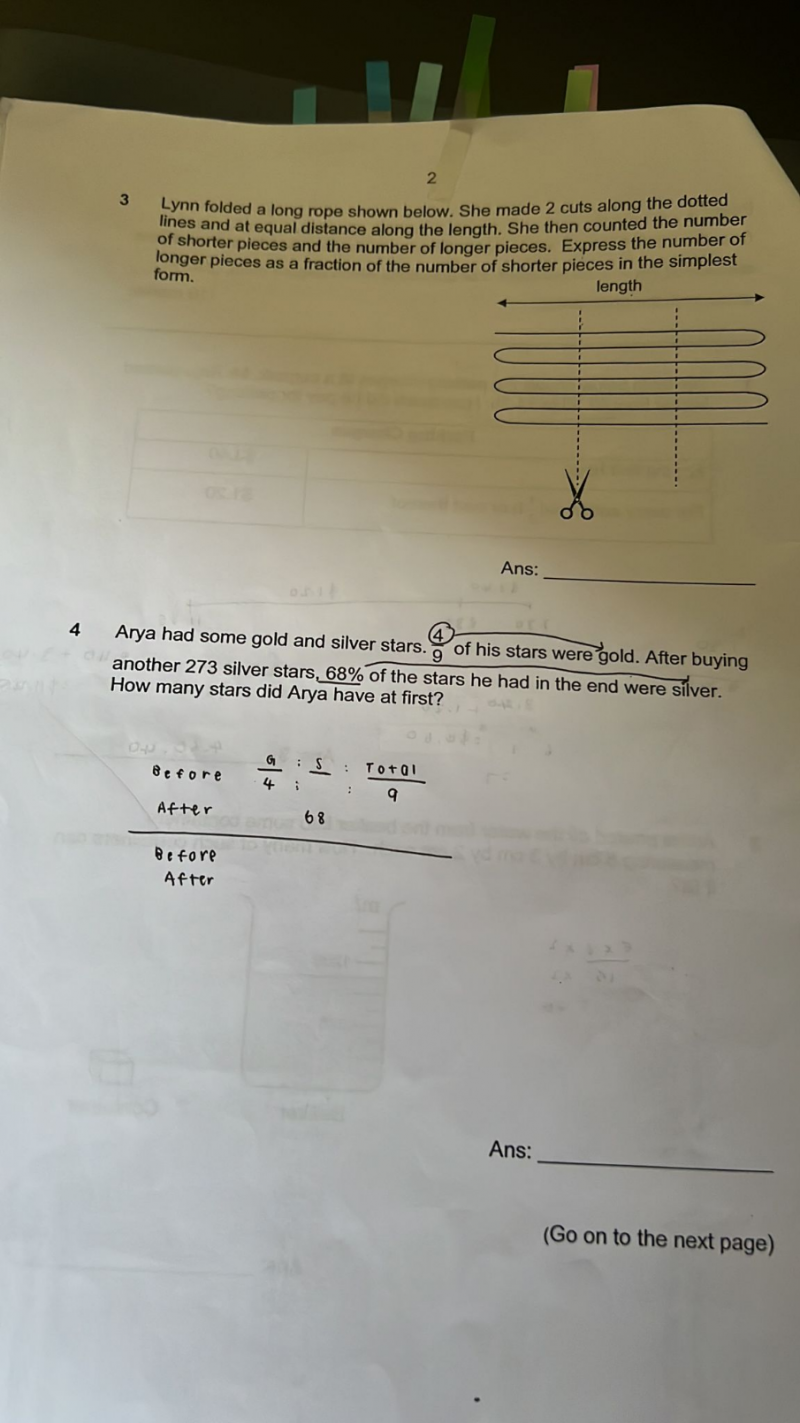# QuestionPls help these two questions thank you.

3.  long strings = 6,   short strings = 9

Therefore, fraction  =  6/9 = 2/3

4.   Gold stars         [u][u][u][u]                          At first, total = 9u

Silver stars       [u][u][u][u][u]          New total = 9u + 273

For the new total, 68% silver means 32% gold, So

4u = 0.32(9u + 273)

4u = 2.88u + 87.36

1.12u = 87.36

u = 78

Number of stars at first = 9u = 9×78 = 702

0 Replies 2 Likes ✔Accepted Answer